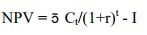# Internal Rate of Return

The discount rate at which the net present value of an investment is equal to zero. The internal rate of return is a time value of money metric, representing the true annual rate of earnings on an investment. In real estate practice, IRR is used together with other return metrics such as equity multiple, cash-on-cash return, and average rate of return to compare real estate investments and make investment decisions.

Unlevered IRR or unleveraged IRR is the internal rate of return of a string of cash flows without financing.

Levered IRR or leveraged IRR is the internal rate of return of a string of cash flows with financing included.The Internal Rate of Return is arrived at by using the same formula used to calculate net present value (NPV), but by setting net present value to zero and solving for discount rate r. In Excel, IRR can be calculated by using the IRR(), XIRR(), or MIRR functions.

« Back to Glossary Index